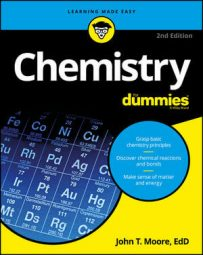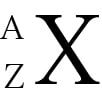##### Chemistry For DummiesDuring the first year of high school chemistry or the first semester of college chemistry, all the terms, units of measurement, and atoms, molecules, elements, and compounds may seem a bit overwhelming. The good news: Your Chem I class doesn't have to be torture. This handy Cheat Sheet provides some basic information you can refer to regularly to make your chemistry class just a tiny bit easier.

## Chemical bonding basics

In bonding, atoms lose, gain, or share electrons in order to have the same number of electrons as the noble gas that’s nearest on the periodic table. Ionic, covalent, and metallic bonds are formed by combinations of metals and nonmetals.

• Metal + nonmetal = ionic bond

• Nonmetal + nonmetal = covalent bond

• Metal + metal = metallic bond

When two elements engage in ionic bonding, one or more electrons are transferred from the metal to the nonmetal, forming ions (charged atoms). The metal, having lost one or more electrons, forms a cation, an ion with a positive charge; the nonmetal, having gained one or more electrons, becomes an anion, an ion with a negative charge.

When two elements form a covalent bond, one or more electron pairs are shared between these two elements. In metallic bonding, which occurs in metals (either a pure metal or an alloy of two or more metals), the valence (outer shell) electrons are donated to a “sea of electrons.”

## Chemistry concepts: energy levels and orbitals

A lot of chemistry is explained by the sharing and trading of electrons between atoms. Understanding how electrons are arranged in an atom is a building block of Chem I.

Electrons in an atom are contained in specific energy levels (1, 2, 3, and so on) that are different distances from the nucleus. The larger the number of the energy level, the farther it is from the nucleus. Electrons that are in the highest energy level are called valence electrons. Within each energy level is a volume of space where specific electrons are likely to be located. These spaces, called orbitals, are of different shapes, denoted by a letter (s, p, d, f, g). (In most cases, only the electrons contained in the s and p orbitals are considered valence electrons.) Electrons seek the lowest energy level possible.

The following electron-filling pattern indicates how the electrons fill into the energy levels. Knowing this pattern is useful in many aspects of chemistry, including predicting the bonding situation of a particular atom and in the prediction of the geometry of a covalent compound.

Electron filling pattern: 1s, 2s, 2p, 3s, 3p, 4s, 3d, 4p, 5s, 4d, 5p, 6s, 4f, 5d, 6p, 7s, 5f

## Digging the mole concept in chemistry

The mole (abbreviate mol and sometimes called Avogadros number) is a conversion number that allows a chemist or chemistry student to move from the microscopic world of atoms, ions, and molecules to the macroscopic world of grams, kilograms, and tons. The mole conversion is used in reaction stoichiometry to predict how much product can be made from a certain amount of reactant or how much reactant is required to produce a certain amount of product.

If you know the particles, moles, or grams of a substance, you can calculate the other two measurements by using the following equation:

1 mole = 6.022 × 1023 particles/mol = formula weight expressed in grams

## Identifying isotopes through representations

In Chem I, you may have to identify isotopes, which are atoms of the same element that have different numbers of neutrons. The following representation allows you to identify a specific isotope of an element. It is used extensively in balancing nuclear reactions.X = element symbol

Z = atomic number (number of protons)

A = mass number (number of protons + number of neutrons)

## Common measurement conversions for chemistry

In order to succeed in your Chem I class, you need to have a firm understanding of basic chemistry measurements and how to convert them from one measurement to another. Following are some important conversions of temperature, size, and pressure as well as metric prefixes to memorize for your chemistry class:

Temperature conversions:

• °F = 9/5(°C) + 32

• °C = 5/9(°F – 32)

• K = °C + 273

English/metric conversions:

• 1 in = 2.54 cm

• 1 lb = 454 g

• 1 qt = 0.946 L

Pressure conversion:

• 1atm = 760 mmHg = 760 torr

Common metric prefixes:

• milli- = 0.001

• centi- = 0.01

• kilo- = 1,000

## The basic chemistry of acids and bases

A lot of chemistry requires you to understand the difference between acids and bases. An acid is a substance that donates an H+ ion to another chemical species called a base. A base is a substance that accepts (combines with) an H+ ion.

If you need to know the concentration of the H+ ion in solution, you can do so by representing the H+ molarity, [H+]. Another way to represent the H+ concentration is the pH, which is the negative logarithm of the H+ molarity. The following equation shows this mathematical relationship as well as the way to calculate the H+ molarity given the pH:

pH = –log[H+]; [H+] = 10–pH

• pH = 7 is neutral.

• pH less than 7 is acidic.

• pH greater than 7 is basic.

## The combined gas law and ideal gas law

When studying the properties of gases, you need to know the relationships between the variables of volume (V), pressure (P), Kelvin temperature (T), and the amount in moles (n) so that you can calculate missing information (P, V, T, or n) and solve reaction stoichiometry problems. Although the pairs of variables have individual relationships, the two most important and useful gas laws are the combined gas law and the ideal gas law:

 Combined gas law (P1V1)/T1 = (P2V2)/T2 (T must be in Kelvin) Ideal gas law PV = nRT (R = 0.0821 L atm/K.mol)